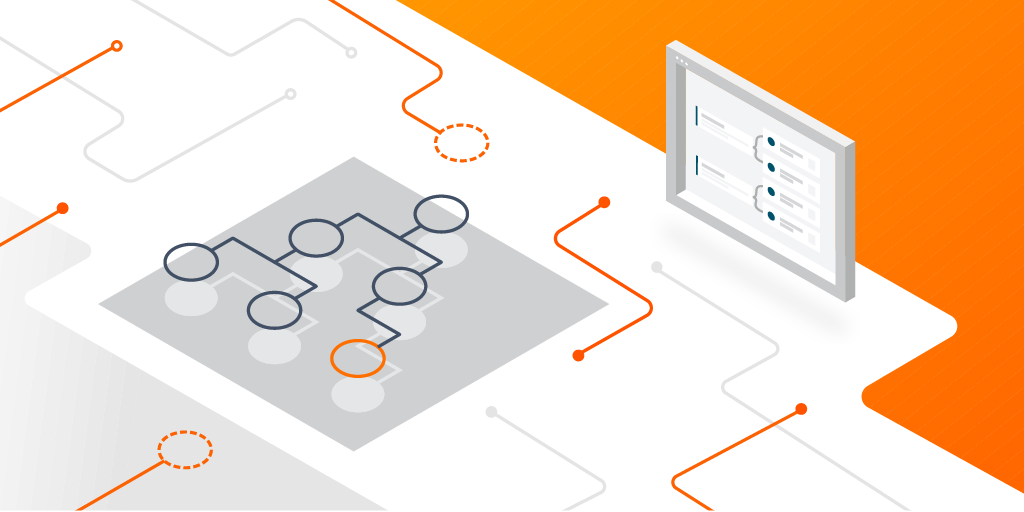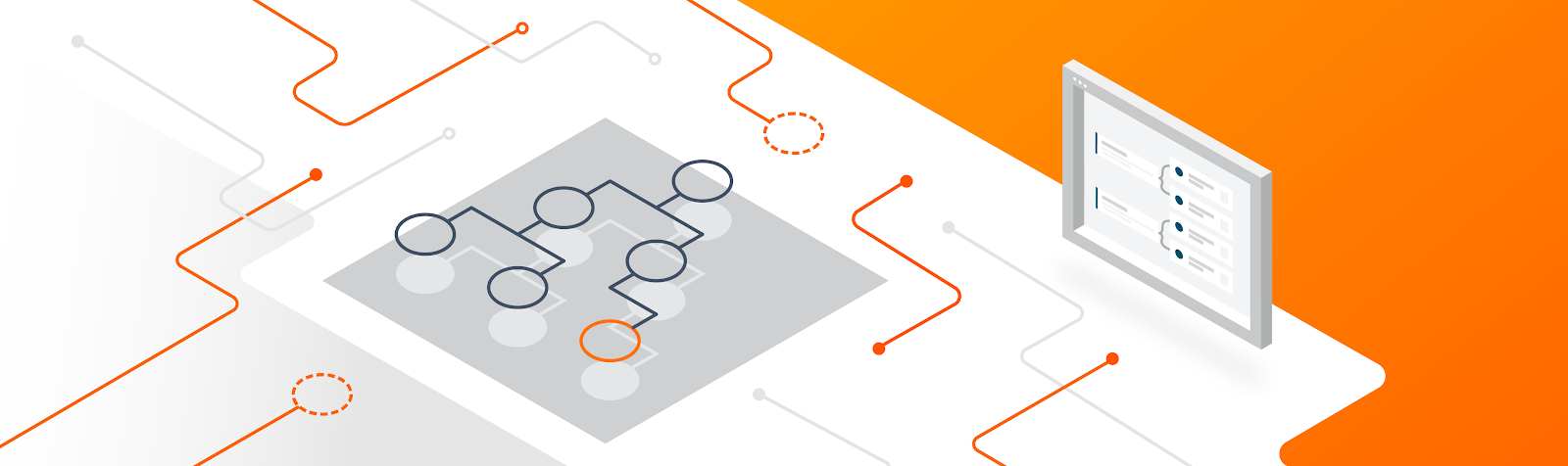Training tree-based models with TensorFlow in just a few lines of code
agosto 03, 2022A guest post by Dinko Franceschi, Broad Institute of MIT and Harvard

Kaggle has become the go-to place to practice data science skills and participate in machine learning model-building competitions. This tutorial will provide an easy-to-follow walkthrough of how to get started with a Kaggle notebook using TensorFlow Decision Forests. It’s a library that allows you to train tree-based models (like random forests and gradient-boosted trees) in TensorFlow.

Why should you be interested in decision forests? There are roughly two types of Kaggle competitions - and the winning solution (neural networks or decision forests) depends on the kind of data you’re working with.

If you’re working with a tabular data problem (these involve training a model to classify data in a spreadsheet which is an extremely common scenario) - the winning solution is often a decision forest. However, if you’re working with a perception problem that involves teaching a computer to see or hear (for example, image classification), the winning model is usually a neural network.

Here’s where the good news starts. You can implement a decision forest in TensorFlow with just a few lines of code. This relatively simple model often outperforms a neural network on many Kaggle problems.

We will explore the decision forests library with a simple dataset from Kaggle, and we will build our model with Kaggle Kernels which allow you to completely build and train your models online using free cloud compute power - similar to Colab. The dataset contains vehicle information such as cost, number of doors, occupancy, and maintenance costs which we will use to assign an evaluation on the car.

Kaggle Kernels can be accessed through your Kaggle account. If you do not have an account, please begin by signing up. On the home page, select the “Code” option on the left menu and select “New Notebook,” which will open a new Kaggle Kernel.

Once we have opened a new notebook from Kaggle Kernels, we download the car evaluation dataset to our environment. Click “Add data” near the top right corner of your notebook, search for “car evaluation,” and add the dataset.

Now we are ready to start writing code. Install the TensorFlow Decision Forests library and the necessary imports, as shown below. The code in this blog post has been obtained from the Build, train and evaluate models with the TensorFlow Decision Forests tutorial which contains additional examples to look at.

``````!pip install tensorflow_decision_forestsimport numpy as np
import pandas
import tensorflow_decision_forests as tfdf``````

We will now import the dataset. We should note that the dataset we downloaded did not contain headers, so we will add those first based on the information provided on the Kaggle page for the dataset. It is good practice to inspect your dataset before you start working with it by opening it up in your favorite text or spreadsheet editor.

``````df = pandas.read_csv("../input/car-evaluation-data-set/car_evaluation.csv")col_names =['buying price', 'maintenance price', 'doors', 'persons', 'lug_boot', 'safety', 'class']
df.columns = col_names
``````

We must then split the dataset into train and test:

``````def split_dataset(dataset, test_ratio=0.30):  test_indices = np.random.rand(len(dataset)) < test_ratio
return dataset[~test_indices], dataset[test_indices]
train_ds_pd, test_ds_pd = split_dataset(df)print("{} examples in training, {} examples for testing.".format(
len(train_ds_pd), len(test_ds_pd)))
``````

And finally we will convert the dataset into tf.data format. This is a high-performance format that is used by TensorFlow to train models more efficiently, and with TensorFlow Decision Forests, you can convert your dataset to this format with one line of code:

``````
train_ds = tfdf.keras.pd_dataframe_to_tf_dataset(train_ds_pd, label="class")
test_ds = tfdf.keras.pd_dataframe_to_tf_dataset(test_ds_pd, label="class")
``````

Now you can go ahead and train your model right away by executing the following:

``````model = tfdf.keras.RandomForestModel()model.fit(train_ds)
``````

The library has good defaults which are a fine place to start for most problems. For advanced users, there are lots of options to choose from in the API doc as random forests are configurable.

Once you have trained the model, you can see how it will perform on the test data.

``````model.compile(metrics=["accuracy"])print(model.evaluate(test_ds))
``````

In just a few lines of code, you reached an accuracy of >95% on this small dataset! This is a simple dataset, and one might argue that neural networks could also yield impressive results. And they absolutely can (and do), especially when you have very large datasets (think: hundreds of thousands of examples, or more). However, neural networks require more code and are resource intensive as they require significantly more compute power.

### Easy preprocessing

Decision forests have another important advantage: there are fewer steps to preprocess the data. Notice in the code above that you were able to pass a dataset with both categorical and numeric values directly to the decision forests. You did not have to do any preprocessing like normalizing numeric values, converting strings to integers, and one-hot encoding them. This has major benefits. It makes decision forests simpler to work with (so you can train a model quickly), and there is less code that can go wrong.

Below, you will see some important differences between the two techniques.

### Easy to interpret

A significant advantage of decision forests is that they are easy to interpret. While the pipeline for decision trees differs significantly from that of training neural networks, there are major advantages for selecting these models for a given task. This is because feature importance is particularly straightforward to determine with decision forests (ensemble of decision trees). Notably, the TensorFlow Decision Forests library makes it possible to visualize feature importance with its model plotter function. Let’s see below how this works!

``tfdf.model_plotter.plot_model_in_colab(model, tree_idx=0)``

We see in the root of the tree on the left the number of examples (1728) and the corresponding distribution indicated by the different colors. Here our model is looking at the number of persons that the car can fit. The largest section indicated by green stands for 2 persons and the red for 4 persons. Furthermore, as we go down the tree we continue to see how the tree splits and the corresponding number of examples. Based on the condition, examples are branched to one of two paths. Interestingly, from here we can also determine the importance of a feature by examining all of the splits of a given feature and then computing how much this feature lowered the variance.

### Decision Trees vs. Neural Networks

Neural networks undoubtedly have incredible representation learning capabilities. While they are very powerful in this regard, it is important to consider whether they are the right tool for the problem at hand. When working with neural networks, one must think a lot about how they will construct the layers. In contrast, decision forests are ready to go out of the box (of course, advanced users can tune a variety of parameters).

Prior to even building a neural network layer by layer, in most cases one must perform feature pre-processing. For example, this could include normalizing the features to have mean around 0 and standard deviation of 1 and converting strings to numbers. This initial step can be skipped right away with Tree-based models which natively handle mixed data.

As seen in the code above, we were able to obtain results in just a few steps. Once we have our desired metrics, we have to interpret them within the context of our problem. Perhaps one of the most significant strengths of Decision Trees is their interpretability. We see in the code above the diagrams that were outputted. Starting at the root, we can follow the branches and quickly get a good idea of how the model made its decisions. In contrast, neural networks are a “black box” that can be difficult to interpret and to explain to a non-technical audience.

### Learning more

If you’d like to learn more about TensorFlow Decision Forests, the best place to start is with the project homepage. You can also check out this previous article for more background. And if you have any questions or feedback, the best place to ask them is on https://discuss.tensorflow.org/ using the tag “tfdf”. Thanks for reading!

Next postA guest post by Dinko Franceschi, Broad Institute of MIT and Harvard Kaggle has become the go-to place to practice data science skills and participate in machine learning model-building competitions. This tutorial will provide an easy-to-follow walkthrough of how to get started with a Kaggle notebook using TensorFlow Decision Forests. It’s a library that allows you to train tree-based models (li…